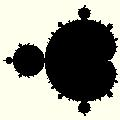# The Mandelbrot Set: Technical Introduction

This article provides the mathematical definition of the Mandelbrot Set and the Julia Set. For a definition that avoids the use of technical language, see Introduction to the Mandelbrot Set.

The Mandelbrot Set is defined by considering the sequence of complex numbers starting z = 0; and proceeding z -> z 2 + c, for each c in the set of all Complex numbers. The Mandelbrot Set is the set of all Complex numbers, c such that the above sequence remains bounded.In theory this is an infinite calculation, just to determine whether one point is inside the Mandelbrot Set or not. It can be proved that once that Modulus of z exceeds 2, then it follows that c is not a member of the Mandelbrot Set – the sequence will tend to Infinity. In this case, we say that c is not a member of the Mandebrot Set, and that c has a “Dwell” value of the number of steps in the sequence to reach this point.

As to when we stop calculating and decide that the sequence will remain bounded forever – we can never know this for sure. Therefore it is necessary to agree a maximum number of iterations, such that if the Modulus of z remains under 2 after this many iterations, we give up. This is known as the “Maximum Dwell”. A value as low as 100 produces a good image of the Mandelbrot Set. For increasing magnifications the Maximum Dwell will need to be increased. (The default setting is for Mandelbrot Explorer to determine an appropriate value for you automatically.)

There is one Julia Set defined for each complex number, c. The Julia Set defined by the number c (known as Jc), is definied by considering the sequence of complex numbers z = a; z -> z 2 + c. If the series is bounded, then a is said to be a member of Julia Set Jc. If not, a is outside that Julia Set.

From this it will become apparent that there is one Julia Set that corresponds to each point within / outside of the Mandelbrot Set. Further, the complex number 0 will be a member of Jc if and only if c is a member of the Mandelbrot Set.

Website Section: